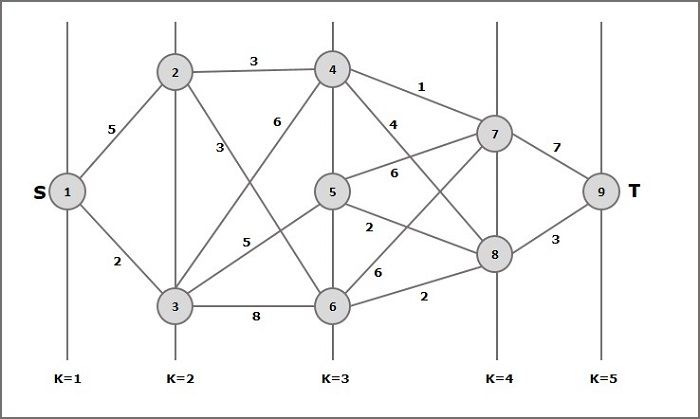• Design and Analysis of Algorithms
• Home

Design and Analysis Multistage Graph

A multistage graph G = (V, E) is a directed graph where vertices are partitioned into k (where k > 1) number of disjoint subsets S = {s1,s2,…,sk} such that edge (u, v) is in E, then u Є si and v Є s1 + 1 for some subsets in the partition and |s1| = |sk| = 1.

The vertex s Є s1 is called the source and the vertex t Є sk is called sink.

G is usually assumed to be a weighted graph. In this graph, cost of an edge (i, j) is represented by c(i, j). Hence, the cost of path from source s to sink t is the sum of costs of each edges in this path.

The multistage graph problem is finding the path with minimum cost from source s to sink t.

Example

Consider the following example to understand the concept of multistage graph.According to the formula, we have to calculate the cost (i, j) using the following steps

Step-1: Cost (K-2, j)

In this step, three nodes (node 4, 5. 6) are selected as j. Hence, we have three options to choose the minimum cost at this step.

Cost(3, 4) = min {c(4, 7) + Cost(7, 9),c(4, 8) + Cost(8, 9)} = 7

Cost(3, 5) = min {c(5, 7) + Cost(7, 9),c(5, 8) + Cost(8, 9)} = 5

Cost(3, 6) = min {c(6, 7) + Cost(7, 9),c(6, 8) + Cost(8, 9)} = 5

Step-2: Cost (K-3, j)

Two nodes are selected as j because at stage k - 3 = 2 there are two nodes, 2 and 3. So, the value i = 2 and j = 2 and 3.

Cost(2, 2) = min {c(2, 4) + Cost(4, 8) + Cost(8, 9),c(2, 6) +

Cost(6, 8) + Cost(8, 9)} = 8

Cost(2, 3) = {c(3, 4) + Cost(4, 8) + Cost(8, 9), c(3, 5) + Cost(5, 8)+ Cost(8, 9), c(3, 6) + Cost(6, 8) + Cost(8, 9)} = 10

Step-3: Cost (K-4, j)

Cost (1, 1) = {c(1, 2) + Cost(2, 6) + Cost(6, 8) + Cost(8, 9), c(1, 3) + Cost(3, 5) + Cost(5, 8) + Cost(8, 9))} = 12

c(1, 3) + Cost(3, 6) + Cost(6, 8 + Cost(8, 9))} = 13

Hence, the path having the minimum cost is 1→ 3→ 5→ 8→ 9.

Useful Video Courses

Video

Compiler Design Online Training

102 Lectures 10 hours

Video

Sequential Circuit Design

30 Lectures 3 hours

Video

Design Patterns Online Training

31 Lectures 4 hours

Video

InDesign Online Training

43 Lectures 1.5 hours

Video

Canva: Become a Graphic Designer

7 Lectures 1 hours

Video

The Ultimate Canva Graphic Design Course

54 Lectures 4 hours